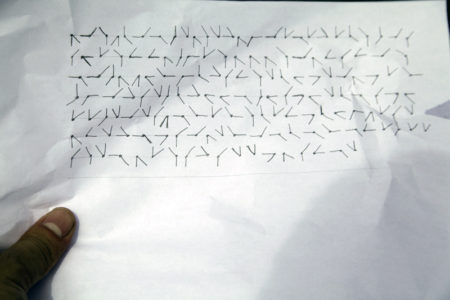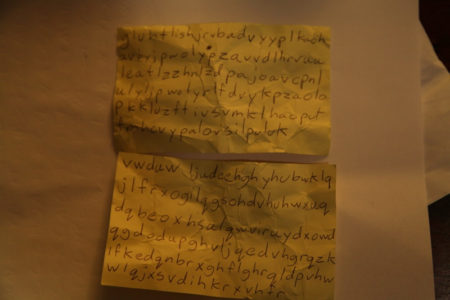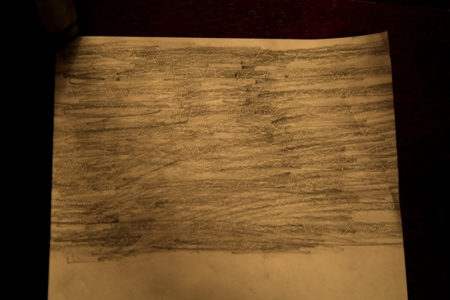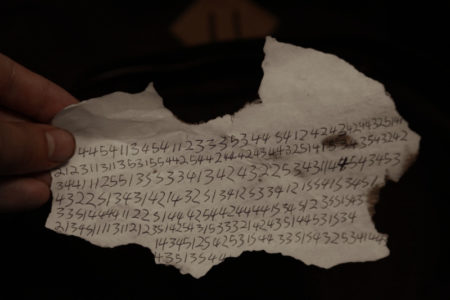## 2014 Crypto Challenge

In 2014, the Khan Academy had a cryptography challenge, called the 2014 Cryptochallenge. It consisted of a narrative leading to clues that were progressively more difficult to decode. The idea was to introduce people unfamiliar with ciphers and cryptography to some basic ciphering methods through examples and clues. I stumbled upon it well after it was debuted, but still had fun going through the challenge.

Please note that while I am displaying the code I wrote to decode the messages, and describe the methodology to arrive at the answers, I will not reveal the decoded messages because I don’t want to ruin the fun for others.

It all starts with the final clue, actually. In the challenge, the goal is to decipher this message that is found:After finding out about a pair of burglars in the vicinity, you find a ciphered communique. The goal is to decode the message by finding clues left behind the pair of villains who communicate using various ciphers. Although this main message is the one we want to decode, we have to work through their previous communications in order to figure out the method of encoding.

## Clue 1The first clue is a simple cipher. Khan Academy gives us the clue that it is a Caeser Cipher, which is where the value of each letter is shifted n places. For example, we could encode the word “CAT” by shifting all the letters 3 values, and end up with “FDW”. Although easy to decode if you know the method, to someone who has no idea, it will just look like gibberish.

This is simple to brute force, as there are only 25 ways the shift the alphabet. I wrote the following C code to decipher the message in all 25 ways, and then I looked through the results for reasonable output.

``````void cipher(const char *input, char *output, int shift) {
for (int i=0 ; i<=strlen(input) ; i++)
if ('A' <= input[i] && input[i] <= 'Z') //Capitals
output[i]=(input[i]-'A'+shift)%26+'A';
else if ('a' <= input[i] && input[i] <= 'z') //Lowers
output[i]=(input[i]-'a'+shift)%26+'a';
else
output[i]=input[i];
}

int main(int argc, char *argv[]) {
const char *messageA="vwduwljudeehghyhubwklqjlfrxogilqgsohdvhuhwxuqdqbeoxhsulqwviruydxowdqgdodupghvljqedvhgrqzklfkedqnbrxghflghrqldpvhwwlqjxsvdihkrxvhfr";

for (int i=1 ; i<=26; i++) {
char outputA[strlen(messageA)];
cipher(messageA, outputA, i);
printf("1/2, Offset: %d\n%s\n\n", i, outputA);

char outputB[strlen(messageB)];
cipher(messageB, outputB, i);
printf("2/2, Offset: %d\n%s\n\n", i, outputB);
}

return 0;
}``````

## Clue 2For this message, they give us the clue of Polyalphabetic Cipher. For this type of cipher, there is a key word that is repeated. Each letter of the alphabet is assigned a value based on it’s order (A=0, B=1… Z=25). The value of the key letter is added to the value of the message letter. If the value is greater than 25, you wrap the value around back to 0 and continue. From Wikipedia, we see this example:

```Plaintext:  ATTACKATDAWN
Key:        LEMONLEMONLE
Ciphertext: LXFOPVEFRNHR
```

A (the 0th letter of the alphabet) plus L (the 11th), when added together would produce 11, which is L.
T (19th) plus E (4th) equals 23, which corresponds to X. Etc.

To reverse the cipher, all you need to do is subtract the values of the keyword from the scrambled text.

The key we will use was alluded to in the decoded first clue. For this clue, the cipher key was doubled; that is each letter of the word was used twice before advancing to the next letter. This is apparently a common practice. What tipped me off is that the thieves previous communication began with START, and ended with END. I presumed that they might do the same with this message. By subtracting the supposed value START from the scrambled message, I could confirm that the keyword was in fact doubled, and then plugged it into the program I wrote to decode the whole message.

``````void cipher(const char *key, const char *input, char *output) {
int n = 0; // Key index

for (int i=0 ; i<strlen(input) ; i++) {
int char_enc = input[i] - 'A'; // Get encoded char value
int char_key = key[n++ % strlen(key)] - 'A'; // get key char value
int char_dec = (26 + char_enc - char_key) % 26; // Reverse vigenere cipher
output[i] = char_dec + 'A'; // Store deciphered message
}
}

int main(int argc, char *argv[]) {
const char *code="SSKKUULLLL"; // Doubled letters for key is common

char output[strlen(message)];
cipher(code, message, output);
printf("%s\n", output);
return 0;
}``````

## Clue 3Clue three was a tough one. I had to look up the hint, which points to a Kahn Academy video called “visual telegraph.” Within the video, we learn of a character encoding method called “Polybius Square.” It explains how characters can be communicated using pairs of numbers that describe a row and column in a character lookup table. Interestingly, in this clue, the message is composed entirely of numbers! We can assume that the table of characters is five by five in size because we never see any numbers smaller than one or larger than five. Additionally, we can also assume that the message starts with the word START again. This can help us figure out the orientation of the lookup table. I made a program to take pairs of numbers and print out the message using the table, and it worked like a charm!

``````void cipher(const char *input, char *output) {
char key={{'A','F','K','P','U'},
{'B','G','L','Q','V'},
{'C','H','M','R','W'},
{'D','I','N','S','X'},
{'E','J','O','T','Y'}};

for (int i=0 ; i<strlen(input)-2 ; i=i+2) {
//Get coordinates from key grid
printf("%c",key[input[i]-'1'][input[i+1]-'1']);

//Save ascii to output
output[i]=key[input[i]-'1'][input[i+1]-'1'];
}
}

int main() {
const char *message="44541134541123335344541242434244325141212311311353155442544244424344325141534354324234411125513553341342432253431144543453432251343142143251341253341215541534513351444411225144425442444415345123551543213451111311212351425431533321424351445315341434512542531544335154325341443043513544";

char output[strlen(message)];
cipher(message, output);
printf("%s\n", output);
return 0;
}``````

## The Final Clue

The final clue stumped me for a while. I ended up getting busy and forgetting about the cryptochallenge. A few months later I found the files on my computer and resumed where I had left off.

Thanks to the previous messages, we found a “safe house” that yielded clues to how this final cipher was put together. Some key insights:

• The message is converted to digits using a method similar to clue 3
• There is a binary key which is obtained from a newspaper clipping where consonants are 0 and vowels are 1
• The symbols represent 2 digits; 1 digit for the vertical/horizontal and 1 digit for the diagonal positions

There were a lot of unknowns, and a few rounds of guess-and-check. One of the key distractions was thinking that each symbol was a letter. That is not the case! From the clues above, we can basically figure out that the process goes like this:

1. Each letter first gets transformed into a 2 digit number using a Polybius Square (like clue 3).
2. From there, the 2 digit number (values maxing out at 66) gets transformed to a 6 bit binary number.
3. The binary number then gets logically XOR’d with the binary numbers produced by the newspaper article. (This is called the pad)
4. The final binary gets split up into 3 bit chunks which get encoded into the final glyphs we found.

To successfully decode the message, we need to reverse the process. Looking at the clues, I made a guess as to what value the glyph positions indicated. After running the program, I didn’t get meaningful output, so I reversed them, and voila, it worked.

This time, I wrote the program in Python since Python is so much more forgiving with types.

The program first takes the pad text (the newspaper article) and converts it into binary.

I converted the glyphs to digits by hand, which was the most time consuming part. I separated the first digit and second digit into two different arrays. Vertical/horizontal lines are called ‘messageA’ and diagonal lines are called ‘messageB’.

I then convert these values sequentially into binary to create a “messagebin” array. Each value in the array gets XOR’d against the corresponding position’s value in the newspaper binary pad array. The result is processed 3 bits at a time and converted into an array of digits.

The list of digits is processed in pairs, and letters are looked up from a table. There was a clue showing that the table of letters was a clockwise spiral starting from the lower left. By adding digits to the spiral at the end, it worked out to fit perfectly in a 6 by 6 arrangement.

After some finagling, the program successfully deciphered the message! I was then able to thwart the would-be criminals!

``````def bin(x):
return ''.join(x & (1 << i) and '1' or '0' for i in range(1,-1,-1))

vowels = ["a","e","i","o","u","y"]

if ord(c) < ord('A') or ord(c) > ord('z'): continue
if c.lower() in vowels :
else:

# Message based on pictograms
#  2 0 3
#   \|/
# 3 - - 1    where straight lines are first digit (A)
#   /|\      and slanted lines are second digit (B)
#  1 2 0

messageA = [2,3,2,2,0,3,3,0,0,2,2,0,3,2,1,3,0,3,0,3,0,0,2,0,3,2,
2,1,0,2,1,1,2,0,1,0,2,2,2,2,2,3,2,1,2,1,3,2,
3,3,0,2,2,1,1,1,0,3,2,1,2,0,0,1,3,0,3,2,3,0,1,
0,1,3,3,2,0,0,0,3,1,1,3,0,1,2,3,1,0,0,3,2,3,1,
0,0,0,0,3,0,2,2,3,1,0,1,2,0,0,1,3,0,1,1,2,0,2,0,
2,1,0,3,3,3,2,3,2,0,1,3,3,0,3,1,3,1,0,0,0,0,
2,2,0,3,2,0,2,2,3,2,0,0,3,2,2,1,3,0]

messageB = [0,3,2,1,0,3,0,1,2,0,2,2,2,0,1,3,3,0,3,2,3,0,2,1,3,3,
3,0,3,2,3,3,0,1,1,3,2,0,0,0,2,3,0,3,3,3,3,2,
0,3,1,0,1,0,2,1,0,2,3,3,2,2,0,0,1,2,3,0,1,3,2,
1,1,3,2,3,2,1,0,2,0,0,0,1,0,3,1,0,2,0,0,3,1,0,
3,3,1,2,3,2,3,1,0,2,3,2,2,0,3,3,1,0,0,1,1,3,3,2,
0,3,2,2,0,1,3,3,0,2,2,3,0,0,0,2,0,3,3,1,3,3,
3,2,2,0,0,3,2,3,2,3,2,2,1,0,3,3,0,2]

# Convert pictogram elements to binary
messagebin = ""
for i in range(len(messageA)):
messagebin += bin(messageA[i])
messagebin += bin(messageB[i])

# XOR pad and message together in binary
charcoords = []
tmp = ""
i = 0
while i < len(padbin) and i < len(messagebin):
# Set length of binary digits here (Probably 3 digit?)
if len(tmp) == 3:
charcoords.append(int(tmp, 2))
tmp = ""
i += 1

# Convert message from binary to alphabet based on Polybius square
key = [["F","G","H","I","J","K"],
["E","X","Y","Z","0","L"],
["D","W","7","8","1","M"],
["C","V","6","9","2","N"],
["B","U","5","4","3","O"],
["A","T","S","R","Q","P"]]

for i in range(0, len(charcoords)-1, 2):
if charcoords[i] > 5 or charcoords[i+1] > 5:
print "?",
else:
print key[charcoords[i]][charcoords[i+1]],
``````

This was a very enjoyable exercise, and taught me some basics about ciphers (and patience). I hope they put on similar exercises in the future!

## Project Euler: 10Problem 10 of Project Euler is to find the sum of all primes under 2,000,000.

This isn’t a hard problem in most languages. I have recently been going through and re-solving problems in various languages for the fun of it. Just for fun, I decided to solve some problems using Bash scripting. Bash has all the constructs of a real programming language, however, the main draw back for problems like this is that it’s slow. VERY slow. Well, lets see what we can do to speed this problem up.

## Brute Force

The brute force approach is pretty simple. Loop through every number, n, less than 2,000,000, and try dividing it by every number from 2 to n-1. If it is divisible, break out of the loop and try the next number.

``````sum=2
for (( i=3; i<2000000; i++ )); do
prime=1
for (( d=2; d<\$i; d++ )); do
if [ \$((\$i % \$d)) -eq 0 ]; then
prime=0
break;
fi
done
if [ \$prime -eq 1 ]; then
sum=\$((\$sum + \$i))
fi
done
echo \$sum
``````

This solution has no optimization, but will still yield a result in a relatively small time-frame for most languages. This would take incredibly long to process primes smaller than 2,000,000. For a benchmark, I have run this through primes less than 2,000, and it took 6.430 seconds. We need to optimize.

## Optimize

There are a lot of simple changes that we can do to get our compute times down. For instance, if we only loop through odd numbers, we have half as many to process.

Another classic technique to optimize a prime test is to check for factors less than or equal to the square root of the number. The reason this works is that if a factor of our number, n, is larger than the square root of n, we will find the other factor has to be smaller than the square root of n, and will thus be detected.

``````sum=2
for (( i=3; i<2000; i+=2 )); do
prime=1
for (( d=2; d*d<=\$i; d++ )); do
if [ \$((\$i % \$d)) -eq 0 ]; then
prime=0
break;
fi
done
if [ \$prime -eq 1 ]; then
sum=\$((\$sum + \$i))
fi
done
echo \$sum``````

These two changes alone bring our compute time down to .319 seconds for primes under 2,000! I still wouldn’t recommend trying computing all primes under 2,000,000. I tried, and killed it after 3 days without a result!

## Sieve of Eratosthenes

Prime sieves are a super fast way of computing prime numbers without having to do any division. This method is attributed to Eratosthenes of Cyrene, a Greek mathematician living about 200 BC. (You can read more about the history on Wikipedia).

Basically, you have an array of every number you want to check. Start by making each element of the array equal to 1, indicating it is a prime.  Go through the array, starting at two. Whenever you hit a value that is 1, mark all the multiples of that value as 0’s. So, we look at element 2, which is prime because it’s value is 1. element whose index is a multiple of 2 should then get marked as 0, since that cannot be prime. You then advance to the next element, and repeat until you’ve visited every number in the array.

``````limit=2000
primes[\$limit]=0
primeList=2
primeIndex=0
sum=0
for (( i=2; i<\$limit; i++ )); do
if [[ \${primes[\$i]} -eq \0 ]]; then
sum=\$((\$sum+\$i))
primeList[\$primeIndex]=\$i
primeIndex=\$((\$primeIndex+1))
for (( n=\$i; n<\$limit; n+=\$i )); do
primes[\$n]=1
done
fi
done

echo \$sum``````

Implementing this method definitely improves results for our benchmark of primes less than 2,000; .186 seconds! However, this approach does not scale up to the desired 2,000,000 because Bash arrays are super slow as they get larger. Even though this approach worked great in our benchmark, it will still take upwards of 3 days to complete for all the primes under 2,000,000.

## Combination of Methods

By using a sieve method combined with our optimized method, I was able to finally get the correct answer in 34 minutes using a Bash script.

I used a sieve to generate the primes between 1 and sqrt(2000000). This was fast since the array wasn’t nearly as big. I then used the array of primes that I generated to check every odd number between 2 and 2,000,000.

Another shortcut to reduce computation is to make a second array containing the squares of each prime. This means that we don’t need to perform multiplication in each loop when we are checking for primality.

``````limit=2000000
sum=0

# Generate primes up to sqrt of 2000000 using sieve
primeLimit=\$(echo "scale=0;sqrt(\$limit*2)" | bc)
primes[\$primeLimit]=0
primeList=2
primeListSquared=4
primeIndex=0
for (( i=2; i<\$primeLimit; i++ )); do
if [[ \${primes[\$i]} -eq \0 ]]; then
sum=\$((\$sum+\$i))
primeList[\$primeIndex]=\$i
primeListSquared[\$primeIndex]=\$i*\$i
primeIndex=\$((\$primeIndex+1))
for (( n=\$i; n<\$primeLimit; n+=\$i )); do
primes[\$n]=1
done
fi
done

# Make sure primeLimit is an odd number
[[ \$((\$primeLimit % 2)) -eq 0 ]] && primeLimit=\$((\$primeLimit + 1))

# Use known primes from above to check each remaining number for primality
for (( i=\$primeLimit; i<\$limit; i+=2 )); do
prime=1
for (( p=0; \${primeListSquared[\$p]}<=\$i; p++ )); do
if [[ \$((\$i % \${primeList[\$p]})) -eq 0 ]]; then
prime=0
break
fi
done
# Record!
if [[ \$prime -eq 1 ]]; then
sum=\$((\$sum+\$i))
fi
done

echo \$sum``````

34 minutes. Finally, a “reasonable” result (by some definitions of reasonable).

## Multi-Processing

The last step I took was by splitting up the task of finding primes into multiple processes. This allowed all 8 of my cores to work on the solution. I moved my prime search method into a function that took a start and stopping point as parameters, and then echoed the sum for that portion back to a while loop that added them all together.

``````limit=2000000

# Generate primes up to sqrt of limit
primeLimit=\$(echo "scale=0;sqrt(\$limit*2)" | bc)
primes[\$primeLimit]=0
primeList=2
primeListSquared=4
primeIndex=0
for (( i=2; i<\$primeLimit; i++ )); do
if [[ \${primes[\$i]} -eq \0 ]]; then
primeList[\$primeIndex]=\$i
primeListSquared[\$primeIndex]=\$i*\$i
primeIndex=\$((\$primeIndex+1))
for (( n=\$i; n<\$primeLimit; n+=\$i )); do
primes[\$n]=1
done
fi
done

function checkRange() {
start=\$1
end=\$2
rangeSum=0

# Make sure range starts at an odd number
[[ \$start -lt 2 ]] && start=3 && rangeSum=2
[[ \$((\$start % 2)) -eq 0 ]] && start=\$((\$start + 1))

# Use known primes to check all remaining numbers for primality
for (( i=\$start; i<\$end; i+=2 )); do
prime=1
for (( p=0; \${primeListSquared[\$p]}<=\$i; p++ )); do
if [[ \$((\$i % \${primeList[\$p]})) -eq 0 ]]; then
prime=0
break
fi
done
# Record!
if [[ \$prime -eq 1 ]]; then
rangeSum=\$((\$rangeSum+\$i))
fi
done
echo \$rangeSum
}

# Split up task into chunks, and check with multiple processes
sum=0
range=\$((\$limit / 50))
for (( start=0; start<\$limit; start+=\$range )); do
checkRange \$start \$((\$start+\$range)) &
done | while read result; do
sum=\$((\$sum+\$result))
echo \$sum
done | tail -1``````

This method brought the compute time down to 3 minutes 22.075 seconds.

## Conclusion

Project Euler gives the guideline that if your solution takes more than a minute, you’re probably doing it wrong. For this particular task though, I think 3 minutes is rather successful given the constraints of Bash. It was a fun project to try to optimize such a relatively simple algorithm within the constraints of this little scripting language.

If you can find any way to speed up the results, please let me know!

Visit the solution on my GitHub page.

## Project Euler 357I recently completed Project Euler 357 after working on it here and there over the course of a couple of days. I decided to do this one out of order because it seemed like a fairly straight foward problem.

Consider the divisors of 30: 1,2,3,5,6,10,15,30.
It can be seen that for every divisor d of 30, d+30/d is prime.

Find the sum of all positive integers n not exceeding 100 000 000
such that for every divisor d of n, d+n/d is prime.

This shouldn’t be hard. Just find the divisors of every number, and see if n+1 is prime. Heck, I already have an isPrime() function written! The brute force approach will be easy to code.

I started off with code resembing this:

``````bool isPrimeGen(int n, bool *primes)
{
for (int d=1; d<=n; d++)
if (n % d == 0)
if (primes[d+n/d] != true)
return false;
return true;
}
int main()
{
int limit = 100000000;
unsigned long long sum = 1;
for (int i=2; i<limit; i++)
if (isPrimeGen(i))
sum += i;
printf("%d", sum)
}``````

Well, this worked… But it took far too long. It took over 4 hours to complete. Obviously this doesn’t fit in with the guidlines that solutions should take less than a minute to complete.

I was able to make a single optimization that got the time down from 4 hours to 12 minutes. A single, tiny change. (It’s actually a similar optimization I made to my prime function when I first started doing these Euler problems…) Instead of looking for every divisor up to the size of n, our number, I put in a square root n, sqrt(n), as the limit for divisor checking.

Another optimization was to make use of looking for patterns in the qualifying numbers.

• n is always even
• n is always 1 less than a prime number
• n/2+2 is always a prime number

Filtering down the potential numbers by using these patterns further reduced the time, but it was still taking longer than I would have liked. I decided to take drastic measures… I pre-computed a ton of primes using a Seive of Eratosthenes.

I made a giant boolean array where any number is marked as a prime or not. This made looking up whether or not primes are numbers super trivial. I’m not sure this is the most elegant solution, but it seemed simple and fast.

``````// Generate primes using sieve
bool *primes = malloc(limit*sizeof(bool));
for (int i=2; i<limit; i++)
primes[i] = true;
primes = false;
primes = false;
for (int i=2; i<limit; i++)
if (primes[i] == true)
{
int n = 2;
while (n*i < limit)
primes[i*n++] = false;
}``````

So, this generates an array, and I pre-load the value for each number as ‘true’ (excepting for 0 and 1 of course). I then go through sequentially, and if I hit a number that is ‘true,’ or prime, every multiple of that number gets marked as ‘false,’ or not prime, until we reach the limit of the array. This is a super fast method for calculating an obscene number of primes very quickly because it doesn’t require any division.

With this pre-loaded table of primes, the code was then refactored to make use of this table rather than my standard isPrime() function.

Putting all these changes together allowed the problem to be solved in under 5 seconds, which is just a tad better than my original 4 hours.

Of course, I won’t reveal the answer here. You’ll need to figure that out on your own. You can, however, check out my full solution on my GitHub account.